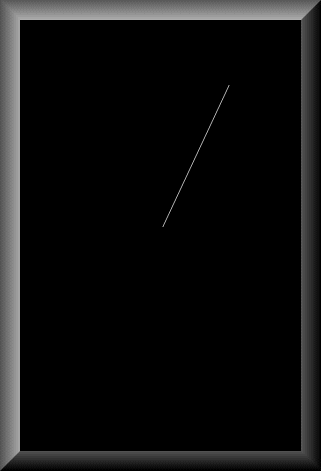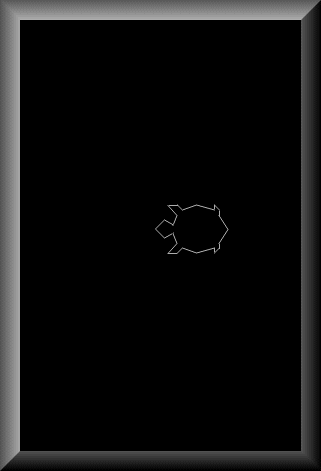Vectrex Programming TOC

Line Drawing

By now you'll probably have noticed, that vectrex programming (using the BIOS) is not all that complicated. Drawing lines follows the same scheme as above examples. We think of some coordinates, put them into appropriate registers and call a function every display round. Drawing figures made of vectors is excactly the same. We keep a list of vector coordinates in memory and again call a function for displaying them. The length of vectors, as allready described above can be varied by two different parameters, the strength of a vector and the time of drawing (scaling).

There are different kinds of vector display functions. Mainly these functions can be sorted by their 'supportiveness'.

1. drawing only one vector
2. drawing a list of vectors
a. including positioning information
b. excluding positioning information
i. including scaling information
ii. excluding scaling information
#1. including brightness information
#2. excluding brightness information
#a. including mode information *
#b. excluding mode information *

* mode means here, that a line can be draw using e.g. patterns and other conditional information

For more information on each function look at the appropriate function describtion in Appendix A. In the following examples I'll first introduce a single line program, and later a program for displaying a vector list.

Following BIOS functions support line drawing:

Draw_Grid_VL (\$FF9F)
Draw_Line_d (\$F3DF)
Draw_Pat_VL (\$F437)
Draw_Pat_VL_a (\$F434)
Draw_Pat_VL_d (\$F439)
Draw_VL (\$F3DD)
Draw_VL_a (\$F3DA)
Draw_VL_ab (\$F3D8)
Draw_VL_b (\$F3D2)
Draw_VL_mode (\$F46E)
Draw_VLc (\$F3CE)
Draw_VLcs (\$F3D6)
Draw_VLp (\$F410)
Draw_VLp_7F (\$F408)
Draw_VLp_b (\$F40E)
Draw_VLp_FF (\$F404)
Draw_VLp_scale (\$F40C)
Mov_Draw_VL (\$F3BC)
Mov_Draw_VL_ab (\$F3B7)
Mov_Draw_VL_a (\$F3B9)
Mov_Draw_VL_d (\$F3BE)
Mov_Draw_VLc_b (\$F3B1)
Mov_Draw_VLcs (\$F3B5)

Now the first example:

Here a screenshot:line1.asm

In the example we assume (correctly) that the Wait_Recal sets DP to D0. First we set a scale factor we want to use for our vector (\$80). As we already know, scale factor is a VIA register, timer1 low byte. Than we set the start coordinate, actually this is a relative coordinate. We know that the Wait_Recal routine sets the beam to the (0,0) position. We use that position as our starting position. NOTE: You must set some starting position with a positioning function. You can not use the (0,0) from Wait_Recal directly. Wait_Recal does some weird stuff, that doesn't allow us to use the positioning given by it directly (when drawing with a standard line function)!

After positioning the vector beam to (0,0) we set the intensity (brightness) to \$5f (reason... see dot plotting :-)). From (Fred's) describtion of the Draw_Line_d routine we know that the routine is actually part of the Draw_VL subroutine, which is used to plot a number of vectors. That number is stored in the Vec_Misc_Count RAM location. Therefor we must insure that only one vector is plotted. We do that by poking 0 to that location

(Note, the BIOS allways ensures this location is 0 before returning, if you don't change that location in your program, than there is no need to clear this location).

Than we set the end position of the vector (relativ to the position we are at now) (the start position is allready known (0,0)). Register A stores the Y position and register B the X position. These positions are the 'translated' strength of the vector. The beam internally is drawn for a fixed amount of time (the scale value) in the direction specified by the Y, X position. The position is internally translated to a voltage with which the electron beam is deflected from the center of the tube.

Anyway, after setting the endposition we are ready to call the 'Draw_Line_d' subroutine. After that we are allready finnished with everything we need to do for drawing a single line, so we go back to the beginning for repainting!

(NOTE:

What strange 'thing' the Wait_Recal does:

It calls right at the end 'Reset0Ref'!

Reset0Ref:

LDD #\$00CC
STB VIA_cntl ;/BLANK low, /ZERO low

...

That means, that both /BLANK AND /ZERO are enabled (0). This means that the vector beam is allways on center (zero). This is true until the VIA_cntl is modified so that the /ZERO flag is high again. The simple 'Draw_Line_d' function does not do this, 'Moveto_d' does however, thus the above construct works ok.

You could instead of the 'Moveto_d' function call also insert the followingtwo lines, which do only the stuff we need:

LDA #\$CE ;/Blank low, /ZERO high
STA VIA_cntl

NOTE 2:

/Blank, /ZERO are both low active.)

The second example:

Now let us draw a couple of lines. Most programs for vectrex will sooner or later require 'combined' line objects. We call these combined lines - vector lists. There are a couple of list types, as you might imagine looking at the above listed sortiment of vector plotting routines. The next example will introduce the most simple form of vector lists. The others are not really much more complicated, but it would be a waste of effort to dig to deeply into them with this document, so they are left out as an exercise for the reader, to go out and explore the BIOS function describtion. Here comes the next example:

Here a screenshot:line2.asm

OK... here we go again. You'll immediatly see, that the program follows the same scheme as all other programs befor. It is nearly the same as the last program. Just one function call is different, with different parameters, and of course the data of the vector list. Therefor I'll from now on only describe the differences to the last program, not the whole program anymore. The new function in this example is Draw_VL (\$F3DD). The function name is a short form of 'Draw Vector List'. This is exactly what it does. It requires just one parameter which is passed in the X register. This is a pointer to the address of the vector list to be drawn. We pass at this stage the address of our list, which is 'turtle_line_list' (the vector list is taken from Vectrex Frogger). The list is very easily constructed, the first byte found at that address is taken as the count, how many vectors make up this list, MINUS 1! (the routine tests for how long the decrement of the count is positive, thus we need a minus 1 here) After the count follow the line 'coordinates'. These 'coordinates' are the same 'vector strength`s' we encountered in the one line version. All of these 'coordinates' are relative to the last relevant position. Thus in the above example the first line will be drawn from 0, 0 (from the Moveto_d, btw the Wait_Recal function) to 2, 2 (equally to the upper right). The next line is drawn from 2, 2 to relative -1, 2. To absolut these coordinates you must add the coordinates. The line`s 'real' coordinates would thus be:

line 1 start 0, 0 end: 2, 2
line 2 start: 2, 2 end: (2-1), (2+2) this is 1, 4
line 3 start: 1, 4 end: (1+2), (4+1) this is 3, 5
...

To visualize that:Ooops, that looks pretty bad, but perhaps you get the point. In the above example you encounter a constant factor in the vector list, here called SPRITE_BLOW_UP. This is just an idea I had at one time to examine the effect of scale factors and vector strengths. Using a defineable constant helps to change the strengths of the vectors very easily. In the above example the vectors actually have strengths of factors of 25 (-125, -100, -75, -50, -25 0, 25, 50, 75, 100, 125). We can pretty easily control the size of our objects without using the scale factor. For the above program I ran a little test. The program as listed above, SPRITE_BLOW_UP = 25 and scale factor of \$10, uses per update round exactly 3158 cycles. If we change the scale factor to \$50 and set the SPRITE_BLOW_UP to 5, which gives us an object of exactly the same size, we need allready 4705 cycles! This does not look like much, but keep in mind, that with just one object you will not be able to create great programs. And that you will also want a pretty fast round, below 30,000 cycles to have a steady image.

Note:

There are also vector list routines, which have a 'mode' or 'pattern' byte. With these routines you are able to draw invisible lines, therefor objects are possible, that are not drawn in one go.

Note:

There are also functions, that provide collision detection of vectorlists. And there are functions with which you can rotate and scale your vectors as need arise. I won't go into these, 'cause they go (IMHO) beyond the scale of a little introduction to vectrex programming. The day you might need them will be the day you don't need an introduction anymore :-).

Vectrex Programming TOC